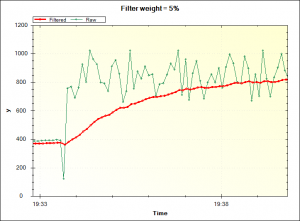The `ExponentialFilter` class implements a simple linear recursive exponential filter for the Arduino. It provides a simple way to smooth noisy measurements from analog sensors without using as much memory as a moving average filter.

Every time you provide a new value (xn), the exponential filter updates a smoothed value (yn):

yn = w × xn + (1 – w) × yn – 1

Here:

• yn is the output of the filter at a moment in time n
• xn is the new input value at a moment in time n
• yn – 1 is the previous output value of the filter
• w is the weighting factor in the range [0, 100]. High values of w (90, for example) favor new data over old data. The output responds quickly to changes in the input but is not smoothed much. Low values of w (10, for example) favor old data over new data. The filter output is heavily smoothed and responds slowly to changes (noisy or not) in the input .

## Functions

The `ExponentialFilter` is a template class that takes a single parameter: the type of measurement to filter. The current filtered value is multiplied by 10 internally to improve precision of the filter. Normally use either `long` (to save code space if you haven’t already used float’s) or `float` (for more accurate results) as the filter parameter.

The constructor takes two arguments:

1. The initial filter weight. This should be between 0 and 100
2. The initial value. Typically 0.

Declare a new exponential filter using:

### Filter(NewValue)

Applies the filter to a new value. See example below.

### SetWeight(NewWeight)

Changes the weight applied when filtering. This value should be between 0 and 100.

High weights (90, for example) favor new data over old data. So, the output responds quickly to changes in the input and is not smoothed much.

Low values of (10, for example) favor old data over new data. So, the output is heavily smoothed and the filter responds slowly to changes (noisy or not) in the input.

### GetWeight()

Returns the current value of the filter weight parameter.

### Current()

Returns the current value of the filter output.

### SetCurrent(NewValue)

Forces the current value to the new value supplied. Typically used to initialize the filter from a measurement.

## Example

This example reads the channel-0 ADC on an Arduino. Both the raw measurement and a heavily filtered value are sent to MegunoLink for plotting.

The noisy output from the analog-to-digital converter (green series) is heavily filtered here (red series):The noisy measurements from the ADC (green) are smoothed by an exponential filter (red)

## Availability

The exponential filter is included in our MegunoLink Arduino Library and can be installed using the Arduino integration tool.

• Matěj Suchánek

Cool! Where can I get it?

• Matěj Suchánek

Nevermind, found it! 😀

• Derek

How do I use this with two separate analog inputs without affecting each other?
Sorry if this is a stupid question but I have little OOP knowledge.

• Paul Martinsen

Hi Derek,

You can just create separate filter variables. For example:

• Ambrus Rácz

Hi!
Can I somehow set with this filter the cutoff frequency? I would like to filter my input signal with a band-pass filter between 20-500 Hz. So e.g. I would use a lowpass filter at 500 Hz, and a highpass at 20 Hz.
Could you help me with this?